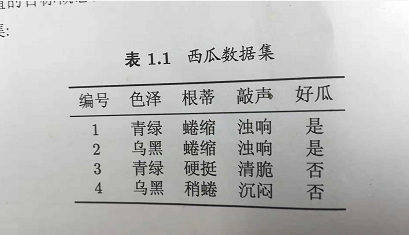2018-12-01 18:12:53 ningbo2016 阅读数 257
• ###### AI时代，机器学习该如何入门？

机器学习入门视频教程，该课程会告诉大家如何入门机器学习，掌握机器学习必要的基础知识，了解机器学习路径。对于机器学习，很多人的观点是：机器学习技术是今后所有技术人员都绕不过的一个门槛。 那么，普通程序员该学习机器学作为一名对机器学习心有向往的程序员，我该以什么样的姿势开始呢？不妨看看该课程。

7368 人正在学习 去看看 CSDN讲师

1.11.2 还没想明白，以后再填坑。。。

2019-04-21 21:13:41 lxfHaHaHa 阅读数 246
• ###### AI时代，机器学习该如何入门？

机器学习入门视频教程，该课程会告诉大家如何入门机器学习，掌握机器学习必要的基础知识，了解机器学习路径。对于机器学习，很多人的观点是：机器学习技术是今后所有技术人员都绕不过的一个门槛。 那么，普通程序员该学习机器学作为一名对机器学习心有向往的程序员，我该以什么样的姿势开始呢？不妨看看该课程。

7368 人正在学习 去看看 CSDN讲师

### 1.1 表1.1中若只包含编号为1，4的两个样例，试给出相应的版本空间

1 青绿 蜷缩 浊响
2 乌黑 稍蜷 沉闷

• 样本空间：所获得的数据集。
• 假设空间：所有可能性的集合。如有 $n$ 个属性，第 $i$ 种属性有 $x_i$ 种选择，那么假设空间的数量就是$\prod _{1}^{n}(x_i+1)+1$种可能，前面的 $(x_i+1)$ 是因为每个属性还存在*（该)属性的泛化取值）这种可能，最后要 $+1$ 是因为存在空集，即没有正例这种情况。
• 版本空间：随样本而定，满足这些样本空间成立的最小集合，即（$样本空间 \leq 版本空间 \leq 假设空间$）。

• 1.色泽=青绿 根蒂=蜷缩 敲声=浊响
• 2.色泽=青绿 根蒂=蜷缩 敲声=沉闷
• 3.色泽=青绿 根蒂=稍蜷 敲声=浊响
• 4.色泽=青绿 根蒂=稍蜷 敲声=沉闷
• 5.色泽=乌黑 根蒂=蜷缩 敲声=浊响
• 6.色泽=乌黑 根蒂=蜷缩 敲声=沉闷
• 7.色泽=乌黑 根蒂=稍蜷 敲声=浊响
• 8.色泽=乌黑 根蒂=稍蜷 敲声=沉闷
• 9.色泽=青绿 根蒂=蜷缩 敲声= *
• 10.色泽=青绿 根蒂=稍蜷 敲声= *
• 11.色泽=乌黑 根蒂=蜷缩 敲声= *
• 12.色泽=乌黑 根蒂=稍蜷 敲声= *
• 13.色泽=青绿 根蒂= * 敲声=浊响
• 14.色泽=青绿 根蒂= * 敲声=沉闷
• 15.色泽=乌黑 根蒂= * 敲声=浊响
• 16.色泽=乌黑 根蒂= * 敲声=沉闷
• 17.色泽= * 根蒂=蜷缩 敲声=浊响
• 18.色泽= * 根蒂=蜷缩 敲声=沉闷
• 19.色泽= * 根蒂=稍蜷 敲声=浊响
• 20.色泽= * 根蒂=稍蜷 敲声=沉闷
• 21.色泽=青绿 根蒂=* 敲声= *
• 22.色泽=乌黑 根蒂=* 敲声= *
• 23.色泽= * 根蒂=蜷缩 敲声= *
• 24.色泽= * 根蒂=稍蜷 敲声= *
• 25.色泽= * 根蒂= * 敲声=浊响
• 26.色泽= * 根蒂= * 敲声=沉闷
• 27.色泽= * 根蒂= * 敲声= *
• 28.空集 Ø

1. 根据编号1的数据可以删除2−8，10−12，14−16，18−20，22，24，26，28
2. 根据编号2的数据可以删除27

• 1.色泽=青绿 根蒂=蜷缩 敲声=浊响
• 9.色泽=青绿 根蒂=蜷缩 敲声= *
• 13.色泽=青绿 根蒂=* 敲声=浊响
• 17.色泽= * 根蒂=蜷缩 敲声=浊响
• 21.色泽=青绿 根蒂=* 敲声= *
• 23.色泽= * 根蒂=蜷缩 敲声= *
• 25.色泽= * 根蒂= * 敲声=浊响

### 1.2 与使用单个合取式来进行假设表示相比，使用“析合范式”将使得假设空间具有更强的表示能力。若使用最多包含k个合取式的析合范式来表达1.1的西瓜分类问题的假设空间，试估算有多少种可能的假设。

2019-04-21 21:10:50 lxfHaHaHa 阅读数 199
• ###### AI时代，机器学习该如何入门？

机器学习入门视频教程，该课程会告诉大家如何入门机器学习，掌握机器学习必要的基础知识，了解机器学习路径。对于机器学习，很多人的观点是：机器学习技术是今后所有技术人员都绕不过的一个门槛。 那么，普通程序员该学习机器学作为一名对机器学习心有向往的程序员，我该以什么样的姿势开始呢？不妨看看该课程。

7368 人正在学习 去看看 CSDN讲师

1. 李宏毅 机器学习入门ppt — 300多页讲述机器学习大概（推荐先看这个，有个整体概念）
2. 西瓜书 机器学习 周志华 — 高清pdf，无封面，带书签，不可复制搜索
3. 李航 统计学 — 机器学习必看

1 第一章 绪论（P19） https://blog.csdn.net/lxfHaHaHa/article/details/89439702
2

2017-10-17 10:20:30 VictoriaW 阅读数 3410
• ###### AI时代，机器学习该如何入门？

机器学习入门视频教程，该课程会告诉大家如何入门机器学习，掌握机器学习必要的基础知识，了解机器学习路径。对于机器学习，很多人的观点是：机器学习技术是今后所有技术人员都绕不过的一个门槛。 那么，普通程序员该学习机器学作为一名对机器学习心有向往的程序员，我该以什么样的姿势开始呢？不妨看看该课程。

7368 人正在学习 去看看 CSDN讲师

7.1 试使用极大似然法估计西瓜数据集3.0中前3个属性的类条件概率。

P(R=r|C=c)=|Dc,r||Dc|

P(S=s|C=c)=|Dc,s||Dc|

P(T=t|C=c)=|Dc,t||Dc|

• 好瓜：

D=8,D=9

• 色泽：
D,绿=3D,绿=3$D_{好瓜,青绿} = 3， D_{坏瓜,青绿}=3$ P(绿|)=38,P(绿|)=39$P(青绿|好瓜)=\frac{3}{8}, P(青绿|坏瓜)=\frac{3}{9}$
D,=4D,=2$D_{好瓜,乌黑} = 4， D_{坏瓜,乌黑}=2$ P(|)=48,P(|)=29$P(乌黑|好瓜)=\frac{4}{8}, P(乌黑|坏瓜)=\frac{2}{9}$
D,=1D,=4$D_{好瓜,浅白} = 1， D_{坏瓜,浅白}=4$ P(|)=18,P(|)=49$P(浅白|好瓜)=\frac{1}{8}, P(浅白|坏瓜)=\frac{4}{9}$
• 根蒂：
D,=5D,=3$D_{好瓜,蜷缩} = 5， D_{坏瓜,蜷缩}=3$ P(|)=58,P(|)=39$P(蜷缩|好瓜)=\frac{5}{8}, P(蜷缩|坏瓜)=\frac{3}{9}$
D,=3D,=4$D_{好瓜,稍蜷} = 3， D_{坏瓜,稍蜷}=4$ P(|)=38,P(|)=49$P(稍蜷|好瓜)=\frac{3}{8}, P(稍蜷|坏瓜)=\frac{4}{9}$
D,=0D,=2$D_{好瓜,硬挺} = 0， D_{坏瓜,硬挺}=2$ P(|)=08,P(|)=29$P(硬挺|好瓜)=\frac{0}{8}, P(硬挺|坏瓜)=\frac{2}{9}$
• 敲声：
D,=6D,=4$D_{好瓜,浊响} = 6， D_{坏瓜,浊响}=4$ P(|)=68,P(|)=49$P(浊响|好瓜)=\frac{6}{8}, P(浊响|坏瓜)=\frac{4}{9}$
D,=2D,=3$D_{好瓜,沉闷} = 2， D_{坏瓜,沉闷}=3$ P(|)=28,P(|)=39$P(沉闷|好瓜)=\frac{2}{8}, P(沉闷|坏瓜)=\frac{3}{9}$
D,=0D,=2$D_{好瓜,清脆} = 0， D_{坏瓜,清脆}=2$ P(|)=08,P(|)=29$P(清脆|好瓜)=\frac{0}{8}, P(清脆|坏瓜)=\frac{2}{9}$

7.2 试证明：条件独立性假设不成立时，朴素贝叶斯分类器仍有可能产生最优贝叶斯分类器。

On the optimality of the simple Bayesian classifier under zero-one loss. 1997.
On Discriminative vs. Generative classifiers: A comparison of logistic regression and naive Bayes. 2002.
The Optimality of Naive Bayes. 2004.

7.3 试编程实现拉普拉斯修正的朴素贝叶斯分类器，并以西瓜数据集3.0为训练集，对p.151 “测1”样本进行判别。

7.4 实践中使用式(7.15)决定分类类别时，若数据维度非常高，则概率连乘di=1P(xi|c)$\prod_{i=1}^dP(x_i|c)$的结果通常会非常接近于0从而导致下溢。试述防止下溢的可能方案。

logP(c)+i=1dlogP(xi|c).

7.5 试证明：二分类任务中两类数据满足高斯分布并且方差相同时，线性判别分析产生贝叶斯最优分类器。

7.6 编程实现AODE分类器，并以西瓜数据集3.0为训练集，对p.151的“测1”样本进行判别。

7.7 给定d个二值属性的二分类任务，假设对于任何先验概率项的估计至少需要30个样例，则在朴素贝叶斯分类器(7.15)中估计先验概率项P(c)$P(c)$需要30×2=60$30\times 2=60$个样例。试估计在AODE(7.23)中估计先验概率P(c,xi)$P(c,x_i)$所需要的样例数（分别考虑最好和最坏情形）。

7.8 考虑图7.3，试证明：在同父结构中，若X1$X_1$的取值已知，则X3$X_3$X4$X_4$独立，若X1$X_1$的取值未知，则X3$X_3$X4$X_4$不独立；在顺序结构中，如果X$X$取值已知，则Y$Y$Z$Z$独立，如果X$X$取值未知，则Y$Y$Z$Z$不独立。(大写表示随机变量，小写表示具体取值)

Bayes网络假设联合概率分布

P(x1,x2,,xn)=i=1nP(xi|pa(xi)).

• X1$X_1$取值未知时，

P(x3,x4)=x1P(x3,x4,x1)=x1P(x3|x1)P(x4|x1)P(x1)P(x3)P(x4)

所以x3$x_3$x4$x_4$不独立。

• X1=x1$X_1=x_1$时，

P(x3,x4|x1)=P(x1,x3,x4)P(x1)=P(x3|x1)P(x4|x1)P(x1)P(x1)=P(x3|x1)P(x4|x1)

所以x3$x_3$x4$x_4$独立。

• 当X未知时，

P(y,z)=xP(x,y,z)=xP(x|z)P(y|x)P(z)P(y)P(z)

所以y和z不独立。

• 当X=x时，

P(y,z|x)=P(x,y,z)P(x)=P(x|z)P(y|x)P(z)P(x)=P(x,z)P(y|x)P(x)=P(y|x)P(z|x)

所以y和z独立。

7.9 以西瓜数据集2.0为例，基于BIC准则构建一个贝叶斯网。

7.10 以西瓜数据集2.0中属性“脐部”为隐变量，试基于EM算法构建一个贝叶斯网。

2018-11-05 16:13:50 weixin_43260483 阅读数 167
• ###### AI时代，机器学习该如何入门？

机器学习入门视频教程，该课程会告诉大家如何入门机器学习，掌握机器学习必要的基础知识，了解机器学习路径。对于机器学习，很多人的观点是：机器学习技术是今后所有技术人员都绕不过的一个门槛。 那么，普通程序员该学习机器学作为一名对机器学习心有向往的程序员，我该以什么样的姿势开始呢？不妨看看该课程。

7368 人正在学习 去看看 CSDN讲师

1.版本空间：
（1）色泽=青绿 根蒂=蜷缩 敲声=浊响
（2）色泽=青绿 根蒂=蜷缩 敲声=*
（3）色泽=青绿 根蒂=* 敲声=浊响
（4）色泽=* 根蒂=蜷缩 敲声=浊响
（5）色泽=青绿 根蒂=* 敲声=*
（6）色泽=* 根蒂=蜷缩 敲声=*
（7）色泽=* 根蒂=* 敲声=浊响
2.析合范式即多个合取式的析取。

k=1时，共48种
k=18时，共1种
k取中间值，比较复杂。
3.（1）最简单的设计：训练样本一致特征越多越好，即一致性比例越高越好，为归纳偏好。考虑归纳偏好应尽量与问题相匹配，所以可使归纳偏好与噪声分布相匹配。
（2）通常认为两个数据的属性越相近，则更倾向于将他们分为同一类。若相同属性出现了两种不同的分类，则认为它属于与他最临近几个数据的属性。也可以考虑同时去掉所有具有相同属性而不同分类的数据，留下的数据就是没误差的数据，但是可能会丢失部分信息。
5.消息推送，如广告推荐；网站排名；图片搜索。